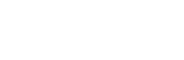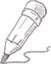#select t.lesson_id,

group_concat(if(排序=1,stu_name,null),score) 第一名,

group_concat(if(排序=2,stu_name,null),score) 第二名,

group_concat(if(排序=3,stu_name,null),score) 第三名

from

(select ts.stu_id, ts.lesson_id, score, stu_name, lesson_name,

dense_rank() over(partition by ts.lesson_id order by score) 排序

from t_score ts

left join t_stu_profile tsp

on ts.stu_id=tsp.stu_id

left join t_lesson tl

on ts.lesson_id=tl.lesson_id) t

group by t.lesson_id;

select

lesson_name,

group_concat(if(排名=1,concat(stu_name,'+',score),null)) as 第一名,

group_concat(if(排名=2,concat(stu_name,'+',score),null)) as 第二名,

group_concat(if(排名=3,concat(stu_name,'+',score),null)) as 第三名

from

(select

lesson_name,

score,

stu_name,

dense_rank() over(partition by t_score.lesson_id order by score desc) as 排名

from t_score

left join t_lesson

on t_lesson.lesson_id=t_score.lesson_id

left join t_stu_profile

on t_stu_profile.stu_id=t_score.stu_id) as t

group by lesson_name;30.6440 6 0 关注作者 收藏

## 评论(0)